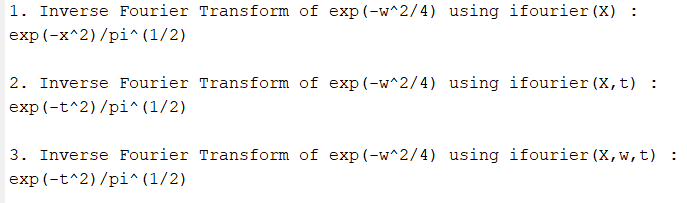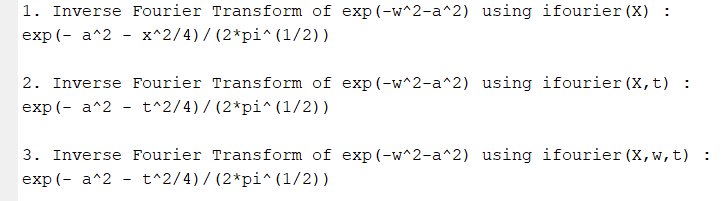# Inverse Fourier transform in MATLAB

• Difficulty Level : Medium
• Last Updated : 30 May, 2021

Inverse Fourier Transform helps to return from Frequency domain function X(ω) to Time Domain x(t). In this article, we will see how to find Inverse Fourier Transform in MATLAB.

The mathematical expression for Inverse Fourier transform is:In MATLAB, ifourier command returns the Inverse Fourier transform of given function. Input can be provided to ifourier function using 3 different syntax.

• ifourier(X): In this method, X is the frequency domain function whereas by default independent variable is w (If X does not contain w, then ifourier uses the function symvar) and the transformation variable is x.
• ifourier(X,transvar): Here, X is the frequency domain function whereas transvar is the transformation variable instead of x.
• ifourier(X,indepvar,transvar): In this syntax, X is the frequency domain function whereas indepvar is the independent variable and transvar is the transformation variable instead of w and x respectively.

Example:

Find the Inverse Fourier Transform of## Matlab

 `% MATLAB code specify the variable``% w and t as symbolic ones``syms w t`` ` `% define Frequency domain function X(w)``X=exp(-w^2/4);`` ` `% ifourier command to transform into``% time domain function x(t)``% using 1st syntax, where by default ``% independent variable = w``% and transformation variable is x .``x1 = ifourier(X);`` ` `% using 2nd syntax, where transformation variable = t``x2 = ifourier(X,t);`` ` `% using 3rd syntax, where independent variable ``% = w (as there is no other``% variable in function) and transformation ``% variable = t ``x3 = ifourier(X,w,t);`` ` `% Display the output value``disp(``'1. Inverse Fourier Transform of exp(-w^2/4) using ifourier(X) :'``)``disp(x1);`` ` `disp(``'2. Inverse Fourier Transform of exp(-w^2/4) using ifourier(X,t) :'``)``disp(x2);`` ` `disp(``'3. Inverse Fourier Transform of exp(-w^2/4) using ifourier(X,w,t) :'``)``disp(x3);`

Output:Example:

Find the Inverse Fourier Transform of## Matlab

 `% MATLAB code to specify the variable %``% a w and t as symbolic ones %``syms a w t`` ` `% define Frequency domain function X(w)``X=exp(-w^2-a^2);`` ` `% ifourier command to transform into``% time domain function x(t)``% using 1st syntax, where by default``% independent variable = w``% and transformation variable is x .``x1=ifourier(X);`` ` `% using 2nd syntax, where transformation ``% variable = t``x2=ifourier(X,t);`` ` `% using 3rd syntax, where independent ``% variable = w (as there is no other``% variable in function) and transformation ``% variable = t ``x3=ifourier(X,w,t);`` ` `% Display the output value``disp(``'1. Inverse Fourier Transform of exp(-w^2-a^2) using ifourier(X) :'``)``disp(x1);`` ` `disp(``'2. Inverse Fourier Transform of exp(-w^2-a^2) using ifourier(X,t) :'``)``disp(x2);`` ` `disp(``'3. Inverse Fourier Transform of exp(-w^2-a^2) using ifourier(X,w,t) :'``)``disp(x3);`

Output:My Personal Notes arrow_drop_up